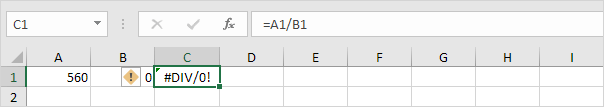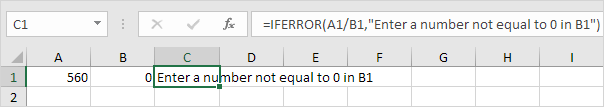# How to use IfError Formula using ExcelThis example illustrates the IFERROR function in Excel.

1. For example, Excel displays the #DIV/0! error when a formula tries to divide a number by 0.2. Use the IFERROR function. If a cell contains an error, an empty string (“”) is displayed.Credit: http://www.excel-easy.com/examples/iferror.html